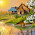## Saturday, June 18, 2011

### Interfacing a DS18S20 with an AVR

This can be a complete project on its own - a simple DIY digital thermometer with LCD display and only a handful of parts - ATMEGA88, DS18S20 and only a resistor running off a regulated 5v supply.

Display type - LCD (can be 16x1, 16x2 or anything larger)
Controller: ATMEGA88
Programming Language: BASIC
Compiler: mikroBASIC PRO for AVR

'''''''''''''''''''''''''''''''''''''''''''''''''''''''''''''''''''''''''''''''''''''''''''''''''''''''''''''''''''''''''''''''''''''''''''''''''''''''''''''''''''
program Temp_DS1820

'Microcontroller: ATmega88 (Atmel AVR)
'Programming Language: BASIC
'Compiler: mikroBASIC PRO for AVR v2.10
'Sensor: DS18S20
'Programmer: Syed Tahmid Mahbub

dim LCD_RS as sbit at PORTB2_bit
dim LCD_EN as sbit at PORTB3_bit
dim LCD_D4 as sbit at PORTB4_bit
dim LCD_D5 as sbit at PORTB5_bit
dim LCD_D6 as sbit at PORTB6_bit
dim LCD_D7 as sbit at PORTB7_bit

dim LCD_RS_Direction as sbit at DDB2_bit
dim LCD_EN_Direction as sbit at DDB3_bit
dim LCD_D4_Direction as sbit at DDB4_bit
dim LCD_D5_Direction as sbit at DDB5_bit
dim LCD_D6_Direction as sbit at DDB6_bit
dim LCD_D7_Direction as sbit at DDB7_bit

sub procedure ConversionDelay()
delay_ms(800)
end sub

sub procedure StabilizeDelay()
delay_ms(200)
end sub

dim Temperature as word
dim TempH as byte
dim TempL as byte
dim TLow as byte
dim vDisp as string
dim DecimalPoint as byte

main:
DDRC = \$FE 'RC0 input for One-Wire
LCD_Init()
LCD_Cmd(_LCD_CURSOR_OFF) 'LCD Cursor off
LCD_Cmd(_LCD_CLEAR) 'Clear LCD
StabilizeDelay() 'Wait for sensor and LCD to stabilize
vDisp = "+124.5 'C"
LCD_Out(1, 1, "Temp:")
while true
OW_Reset(PORTC, 0) 'Reset command to initialize One-Wire
OW_Write(PORTC, 0, \$CC) 'Skip ROM Command
OW_Write(PORTC, 0, \$44) 'Convert_T command
ConversionDelay() 'Provide delay for conversion
OW_Reset(PORTC, 0) 'Reset command to initialize One-Wire
OW_Write(PORTC, 0, \$CC) 'Skip ROM Command
Temperature = Temperature + ((OW_Read(PORTC, 0)) << 8) 'Read Temperature high byte and convert low and high bytes to one 16-bit word
TempH = Hi(Temperature) 'High 8 bits of Temperature
TempL = Lo(Temperature) 'Low 8 bits of Temperature
DecimalPoint = Temperature.B0 'Check if Temperature is integer or fractional
if (Temperature and \$8000) then 'If reading is negative
vDisp = "-"
TempL = byte((not TempL + 1) >> 1)
vDisp = "+"
TempL = TempL >> 1 'Shift one position right (divide by 2) to get integer reading and get rid of decimal point
end if
vDisp = TempL div 100 + 48 'Get hundreds and convert to ASCII
vDisp = (TempL div 10) mod 10 + 48 'Get tens and convert to ASCII
vDisp = TempL mod 10 + 48 'Get units and convert to ASCII
if (DecimalPoint) then 'If reading is fractional, ie has 0.5 at end
vDisp = "5"
else 'If reading is a whole number
vDisp = "0"
end if
LCD_Out(1, 8, vDisp) 'Show temperature
wend
end.

http://www.4shared.com/file/WMXzmmO5/DS1820_Temp_LCD.html

The code can be freely used and there is no copyright restrictions or anything as such. Only credit the author (me) where necessary.

1.Hi Tahmind
thanks for the very useful info on avr timer use. Can you help with controlling a single stepper motor with ATMega128 and a gekodrive?
I am having trouble getting it to run fast. I am using C but am quite new to avr. I need to run the stepper forward for a set number of steps (length) only. Many thanks.
Richard (South Africa)

2.Which compiler are you using? Can I see the code you've done so far?

3.@Tahmid: Which schematic capture program are you using? I'm using Eagle 5.11.0 on Ubuntu 11.10.

4.I'm currently using Proteus in Windows.

5.http://www.4shared.com/file/WMXzmmO5/DS1820_Temp_LCD.html
6.